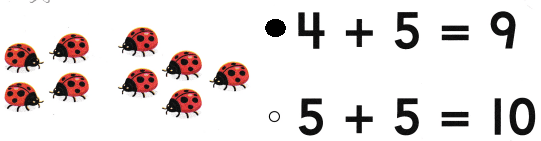# Texas Go Math Kindergarten Module 13 Assessment Answer Key

Refer to our Texas Go Math Kindergarten Answer Key Pdf to score good marks in the exams. Test yourself by practicing the problems from Texas Go Math Kindergarten Module 13 Assessment Answer Key.

## Texas Go Math Kindergarten Module 13 Assessment Answer Key

Concepts and Skills

Question 1.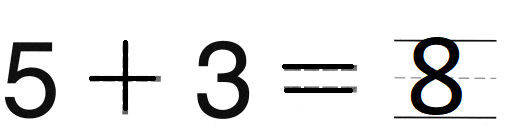Explanation:
The sum of 5 and 3 is 8
There are 5 birds are sitting on a tree
3 more birds joined them
5 + 3 = 8

Question 2.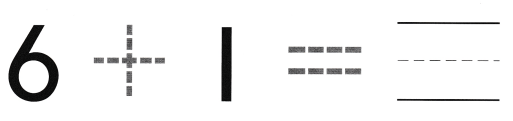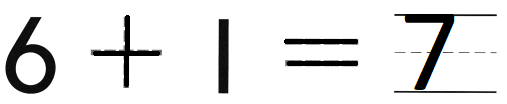Explanation:
the sum of 6 and 1 is 7
6 ducks are swimming in a pond 1 more duck joined them
6 + 1 = 7

Question 3.Explanation:
There are 5 cars and 5 aero planes
5 + 5 = 10
so, there are 10 toys in all.

Directions
1-2. Complete the addition sentence. TEKS K.1.E, K.3.B 3. Keisha has five toy cars and five toy airplanes. How many toys does she have in all? TEKS K.1.A, K.3.B

Question 4.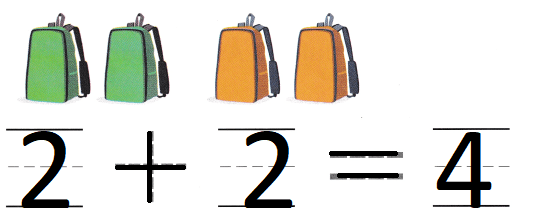Explanation:
There are 2 green bags and 2 orange bags
2 + 2 = 4
so, there are 4 bags.

Question 5.Explanation:
There are 5 blue crayons and 5 yellow crayons
5 + 5 = 10
so, there are 10 yellow crayons.

Question 6.
Texas Test Prep